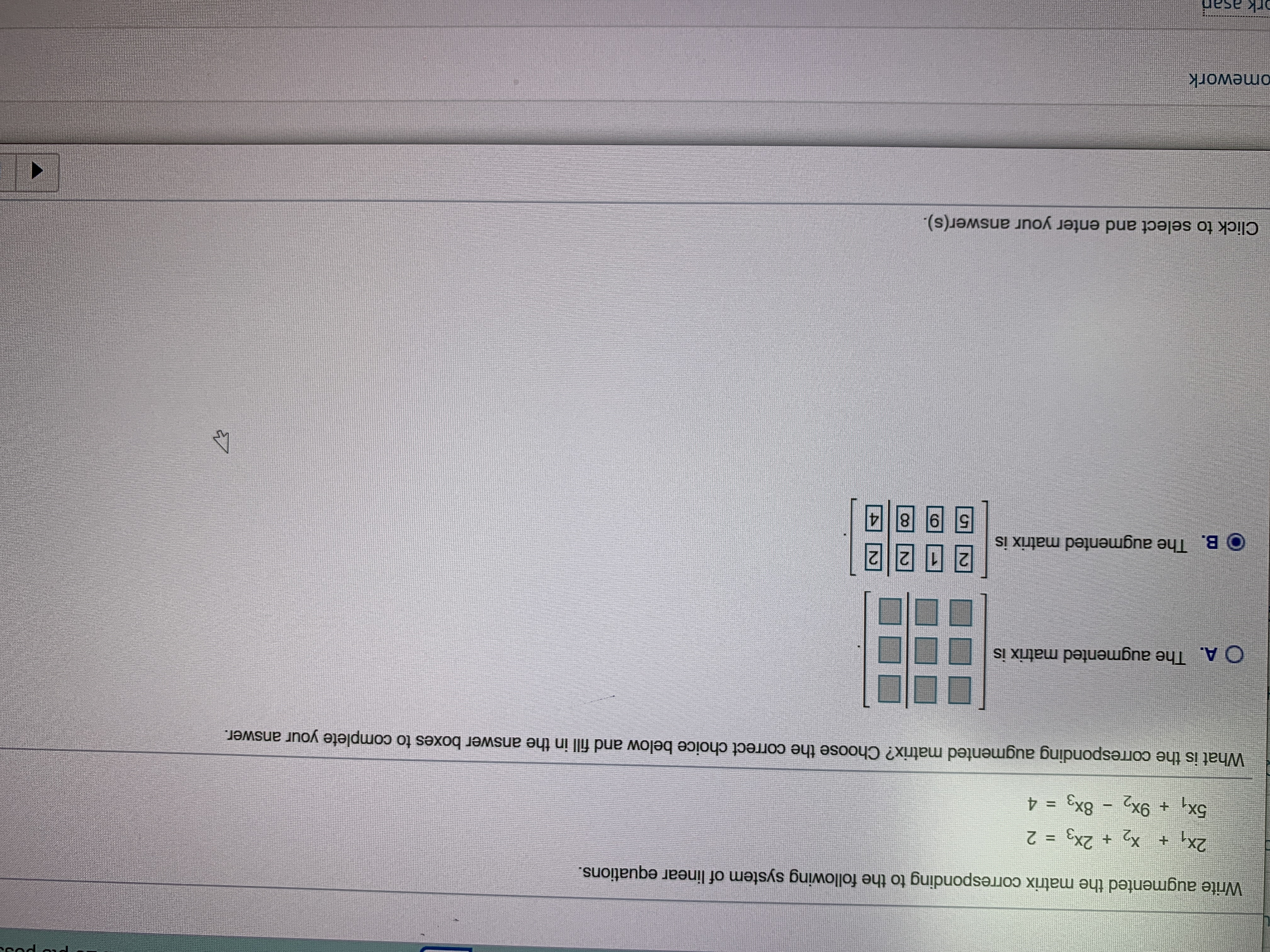# Write augmented the matrix corresponding to the following system of linear equations.2x, ++ 2x3 25x, +9x2 8x3 = 4What is the corresponding augmented matrix? Choose the correct choice below and fill in the answer boxes to complete your answer.O A. The augmented matrix isB. The augmented matrix isВ.5 98Click to select and enter your answer(s).omeworkrk asar

Questionhelp_outlineImage TranscriptioncloseWrite augmented the matrix corresponding to the following system of linear equations. 2x, + + 2x3 2 5x, +9x2 8x3 = 4 What is the corresponding augmented matrix? Choose the correct choice below and fill in the answer boxes to complete your answer. O A. The augmented matrix is B. The augmented matrix is В. 5 98 Click to select and enter your answer(s). omework rk asar fullscreen
check_circleExpert Solution
Step 1

There are two equations with 3 variables given in the question.

Hence, its augmented matrix is a 2 by 4 matrix whose elements of first column are coefficients of x1, elements of second column are coef...

### Want to see the full answer?

See Solution

#### Want to see this answer and more?

Solutions are written by subject experts who are available 24/7. Questions are typically answered within 1 hour*

See Solution
*Response times may vary by subject and question
Tagged in

### Other